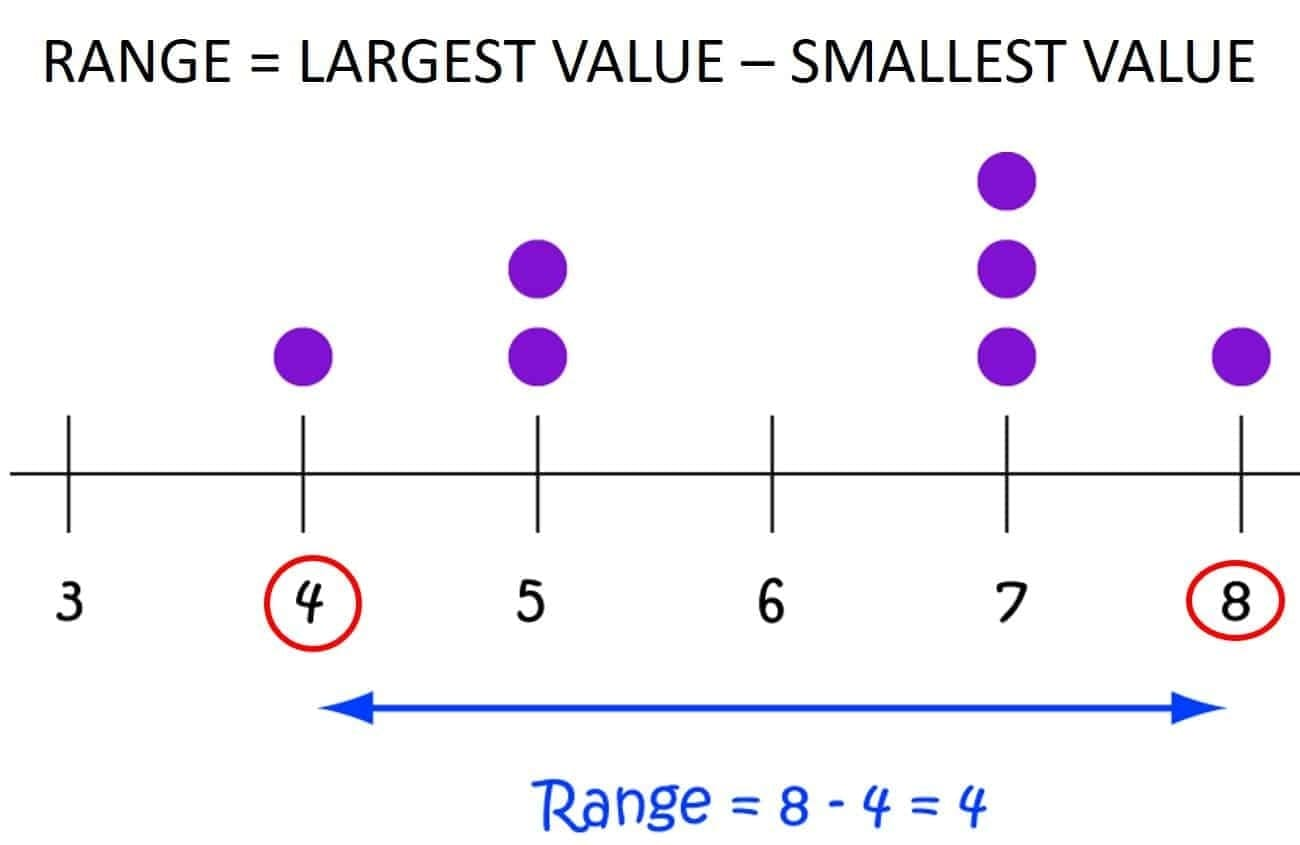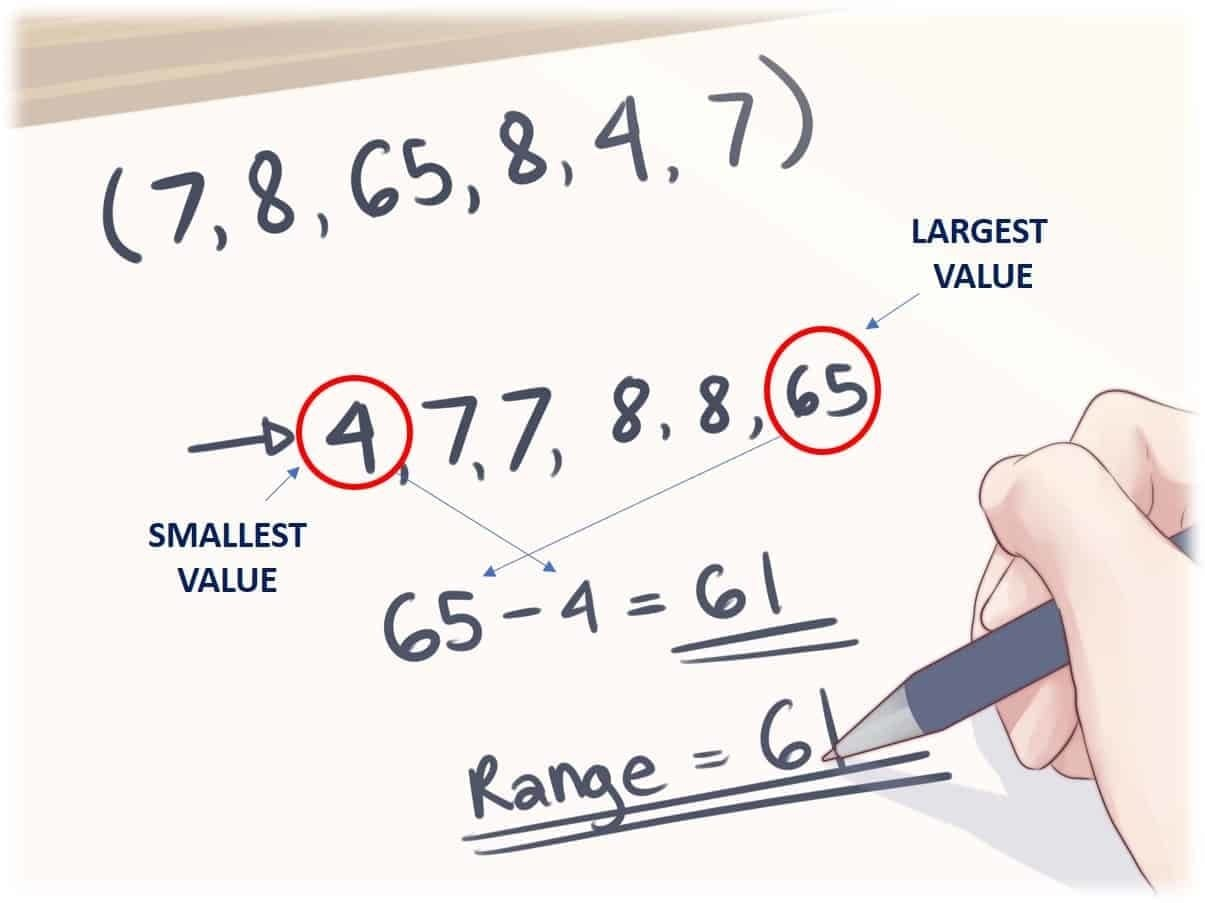Select Page## Using “Range” as a Measure of Data Spread or Dispersion

A measure of dispersion tells you the spread of the data. This is important to know the spread of your data when describing your data set. Most describe a set of data by using only the mean or median leaving out a description of the spread.

There are two main measures of spread or dispersion:

• Range
• Standard Deviation

### In this article we will discuss the Range of the Distribution

The Range is the difference between the largest and the smallest observations in a set of data. It is a rudimentary measure of variability. Range does not give us an idea about the spread of the observations around some central value.### Advantages of using Range as a Measure of Spread or Dispersion

• Range can be calculated easily.
• It is a simplest measure of dispersion.

### Limitations of using Range as a Measure of Spread or Dispersion

• Range is not based on all the observations of the series. It takes into account only the most extreme cases.
• It helps us to make only a rough comparison of two or more groups of variability.
• The range takes into account the two extreme scores in a series.

Thus when the number of data in the data set is small or when there are large enough gaps in the distribution, range is unreliable.as measure of variability.

Do you have an example of using Range as a measure of spread during a Lean Six Sigma project or in a management meeting? If you do, let us know in the comments.LEARN THE 10 SIMPLE STEPS THAT WE TEACH LEAN SIX SIGMA GREEN BELTS TO GET AMAZING RESULTS FROM THEIR PROJECTS.